# Division + natural numbers - math problems

#### Number of problems found: 109

• RemainderWhat is the remainder of the division of natural numbers 293 and 7?
• DivisionWhich number in division 16 give 12 and the rest 3?
• Quotient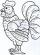Determine the quotient (q) and the remainder (r) from division numbers 100 and 8. Take the test of correctness.
• Twenty-fiveHow many are three-digit natural numbers divisible by 25?
• Count of roots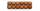How many solutions has equation x. y = 7757 with two unknowns on the set of natural numbers?
• Divisible by nineHow many three-digit natural numbers in total are divisible without a remainder by the number 9?
• Life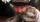Calculate how many years has man lived for 12248 days?
• RemainderA is an arbitrary integer that gives remainder 1 in the division with 6. B is an arbitrary integer that gives remainder 2 the division by. What makes remainder in division by 3 product of numbers A x B ?
• Coloured numbersMussel wrote four different natural numbers with coloured markers: red, blue, green and yellow. When the red number divides by blue, it gets the green number as an incomplete proportion, and yellow represents the remainder after this division. When it div
• Reminder and quotientThere are given the number C = 281, D = 201. Find the highest natural number S so that the C:S, D:S are with the remainder of 1,
• DivisibilityDetermine all divisors of number 84.
• Divisible by fourAre 240 divisible by 4?
• Lcm 2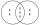Create the smallest possible number that is divisible by numbers 5,8,9,4,3
• Divisors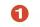Find all divisors of number 493. How many are them?
• Unknown numberIf I reduced the sum of the numbers 70 and the unknown number three times, I would get 100. what is the unknown number?
• Simple sequence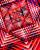Continue with this series of numbers: 1792,448, 112, _, _
• What is 11What is the quotient of Three-fifths and 1 Over 10?
• Drawing from a hatWhen drawing numbers from a hat from 1 to 35, we select random given numbers. What is the probability that the drawn numbers will be divisible by 8 and 2?
• Chocolates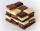In the market we have 3 kinds of chocolates. How many ways can we buy 14 chocolates?
• In the bowlThere are 12 pears in the bowl. Erik ate a third. How many pears remained in the bowl?

Do you have an interesting mathematical word problem that you can't solve it? Submit a math problem, and we can try to solve it.

We will send a solution to your e-mail address. Solved examples are also published here. Please enter the e-mail correctly and check whether you don't have a full mailbox.

Please do not submit problems from current active competitions such as Mathematical Olympiad, correspondence seminars etc...
Do you want to perform natural numbers division - find the quotient and remainder? Division Problems. Natural numbers - math problems.